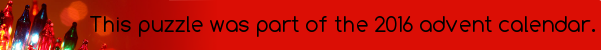mscroggs.co.uk
mscroggs.co.uksubscribe2016's Advent calendar ended with a murder mystery, with each of the murderer, motive, weapon and location being a digit from 1 to 9. Here are the clues:
10
None of the digits of 171 is the location.
3
None of the digits of 798 is the motive.
7
One of the digits of 691 is the location.
16
None of the digits of 543 is the location.
5
One of the digits of 414 is the murderer.
20
The first digit of 287 is the number of false red clues.
8
Clues on days that are factors of 768 are all true.
22
The murderer is the square root of one of the digits of 191.
11
One of the digits of 811 is the weapon.
19
The highest common factor of the weapon and 128 is 1.
13
None of the digits of 512 is the murderer.
18
One of the digits of 799 is the motive.
17
None of the digits of 179 is the motive.
6
None of the digits of 819 is the location.
24
One of the digits of 319 is total number of false clues.
23
One of the digits of 771 is the murderer.
2
The weapon is not one of the digits of 435.
14
The final digit of 415 is the number of true blue clues.
4
The weapon is a factor of 140.
12
The number of false clues before today is the first digit of 419.
9
One of the digits of 447 is the motive.
1
None of the digits of 563 is the motive.
21
One of the digits of 816 is the murderer.
15
One of the digits of 387 is the motive.

## Archive

Show me a random puzzle
▼ show ▼